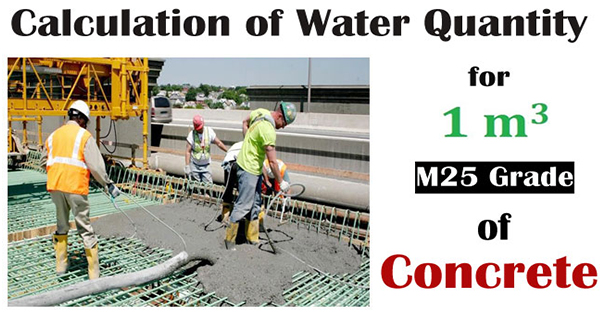### Calculation of water quantity for 1m3 M25 grade of concreteWhile going to build up concrete construction, sufficient amount of water is required. The quantity of water differs according to diverse concrete grades.

In this civil engineering article, the calculation is done to determine the quantity of water for M25 grade of concrete.

Here, the volume of concrete is taken as 1m3.

Initially, it is required to find out the quantity of cement for 1m3 concrete with m25 grade:-

For 1m3 concrete, the quantity of cement required = 9.51 bags

As we know, 1 bag of cement is equivalent to 50 kg

So, for 9.51 bags, the required quantity of cement = 50 x 9.51 = 476 kg

The water–cement ratio means the proportion of the weight of water to the weight of cement utilized in a concrete mix.

The water to cement ratio is measured by dividing the water in one cubic yard of the mix ( in pounds) with the cement in the mix (in pounds). But for workability, additional water is provided which vaporizes in due course of time and departs voids behind. More water cement ratio produces porousness in concrete.

Water–cement ratio is normally retained as 0.45 to 0.60. It is mainly based on the exposure condition of concrete but minimum water cement ratio is 0.38 for full hydration of concrete.

The water is added according to the quantity of cement.

Suppose, 0.5 kg of water is utilized in 1 kg of cement.

The quantity of water is determined as follow:-

= 476 x 0.5 = 238 kg

As water may vaporize, wastage should also be taken into consideration.

Assume the wastage as 5% of total water quantity.

So, 238 kg + 5/100 x 238 = 250 kg